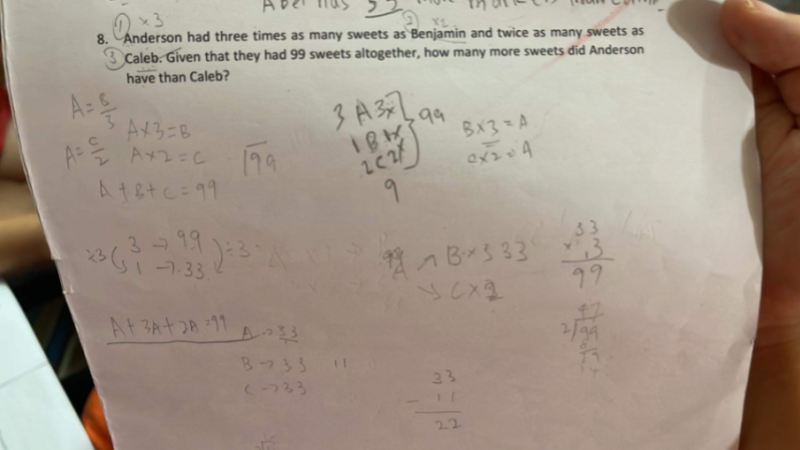# QuestionThank you.

My steps is A:B:C

6:2:3

11units =99

1unit=9

54-27=27

Did I do correct?thanks.

Given

Anderson         :  Benjamin      :           Caleb

3                     :           1          :           3/2= 1.5     multiply by 2 for all ratio gives

6                     :           2          :           3

Total 6+2+3=11

Difference in Anderson and Caleb is 6-3 =3

Given total 99 sweets,

99 * 3/11= 27

Anderson has 27 more sweets than Caleb

0 Replies 2 Likes ✔Accepted Answer